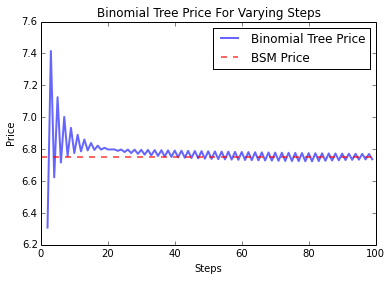Demonstrates how to price European options using QuantLib Python. Methods using Black-Scholes-Merton formula and binomial tree will be discussed.

Visit here for other QuantLib Python examples. If you found these posts useful, please take a minute by providing some feedback.

I have written about option pricing earlier. The introduction to option pricing gave an overview of the theory behind option pricing. The post on introduction to binomial trees outlined the binomial tree method to price options.

In this post, we will use QuantLib and the Python extension to illustrate a very simple example. Here we are going to price a European option using the Black-Scholes-Merton formula. We will price them again using the Binomial tree and understand the agreement between the two.

In :
import QuantLib as ql # version 1.5
import matplotlib.pyplot as plt
%matplotlib inline


Let us consider a European call option for AAPL with a strike price of \$130 maturing on 15th Jan, 2016. Let the spot price be \$127.62. The volatility of the underlying stock is know to be 20%, and has a dividend yield of 1.63%. Lets value this option as of 8th May, 2015.

In :
# option data
maturity_date = ql.Date(15, 1, 2016)
spot_price = 127.62
strike_price = 130
volatility = 0.20 # the historical vols for a year
dividend_rate =  0.0163
option_type = ql.Option.Call

risk_free_rate = 0.001
day_count = ql.Actual365Fixed()
calendar = ql.UnitedStates()

calculation_date = ql.Date(8, 5, 2015)
ql.Settings.instance().evaluationDate = calculation_date


We construct the European option here.

In :
# construct the European Option
payoff = ql.PlainVanillaPayoff(option_type, strike_price)
exercise = ql.EuropeanExercise(maturity_date)
european_option = ql.VanillaOption(payoff, exercise)


The Black-Scholes-Merto process is constructed here.

In :
spot_handle = ql.QuoteHandle(
ql.SimpleQuote(spot_price)
)
flat_ts = ql.YieldTermStructureHandle(
ql.FlatForward(calculation_date, risk_free_rate, day_count)
)
dividend_yield = ql.YieldTermStructureHandle(
ql.FlatForward(calculation_date, dividend_rate, day_count)
)
flat_vol_ts = ql.BlackVolTermStructureHandle(
ql.BlackConstantVol(calculation_date, calendar, volatility, day_count)
)
bsm_process = ql.BlackScholesMertonProcess(spot_handle,
dividend_yield,
flat_ts,
flat_vol_ts)


Lets compute the theoretical price using the AnalyticEuropeanEngine.

In :
european_option.setPricingEngine(ql.AnalyticEuropeanEngine(bsm_process))
bs_price = european_option.NPV()
print "The theoretical price is ", bs_price

The theoretical price is  6.74927181246


Lets compute the price using the binomial-tree approach.

In :
def binomial_price(bsm_process, steps):
binomial_engine = ql.BinomialVanillaEngine(bsm_process, "crr", steps)
european_option.setPricingEngine(binomial_engine)
return european_option.NPV()

steps = range(2, 100, 1)
prices = [binomial_price(bsm_process, step) for step in steps]


In the plot below, we show the convergence of binomial-tree approach by comparing its price with the BSM price.

In :
plt.plot(steps, prices, label="Binomial Tree Price", lw=2, alpha=0.6)
plt.plot([0,100],[bs_price, bs_price], "r--", label="BSM Price", lw=2, alpha=0.6)
plt.xlabel("Steps")
plt.ylabel("Price")
plt.title("Binomial Tree Price For Varying Steps")
plt.legend()

Out:
<matplotlib.legend.Legend at 0x7f0b85fa7510># Conclusion

This post shows how to price European Options using the theoretical and binomial-tree methods in QuantLib Python. You can download the ipython notebook on European option pricing with QuantLib.

quantlib   python   finance   option models

Related PostI am Goutham Balaraman, and I explore topics in quantitative finance, programming, and data science. You can follow me @gsbalaraman.

Checkout my bookUpdated posts from this blog and transcripts of Luigi's screencasts on YouTube is compiled into QuantLib Python Cookbook .